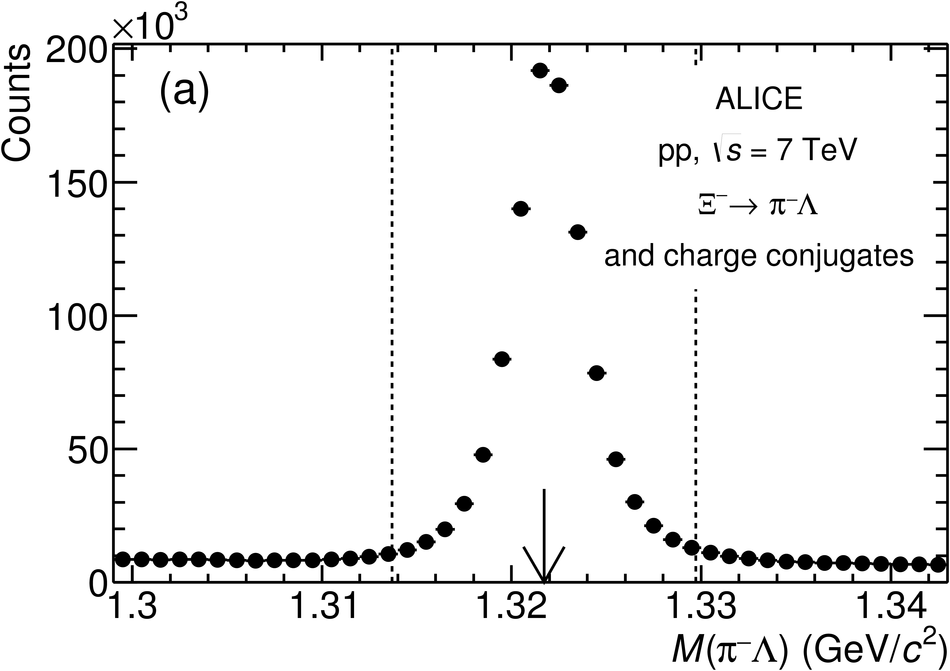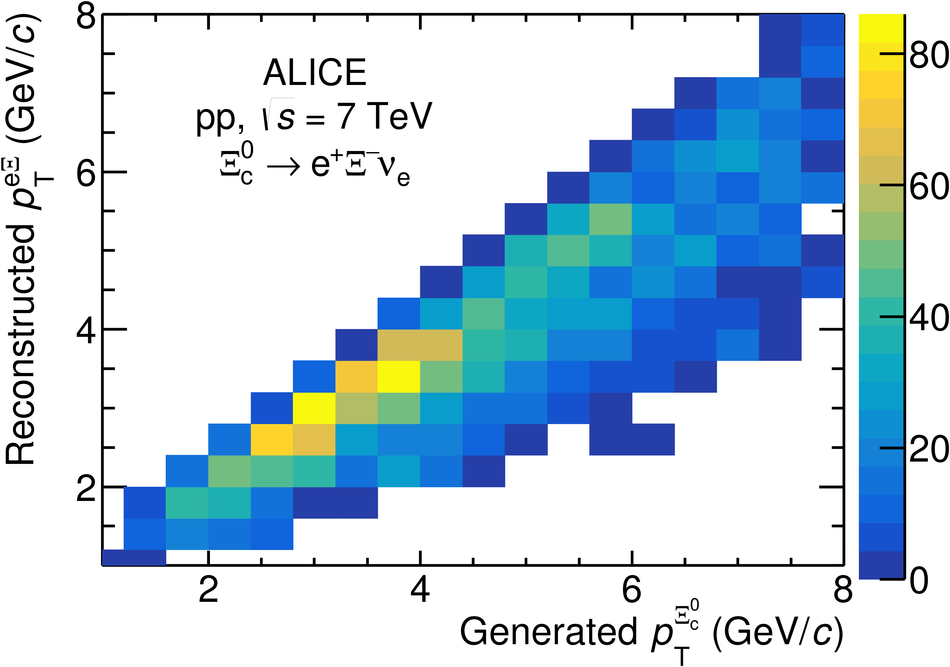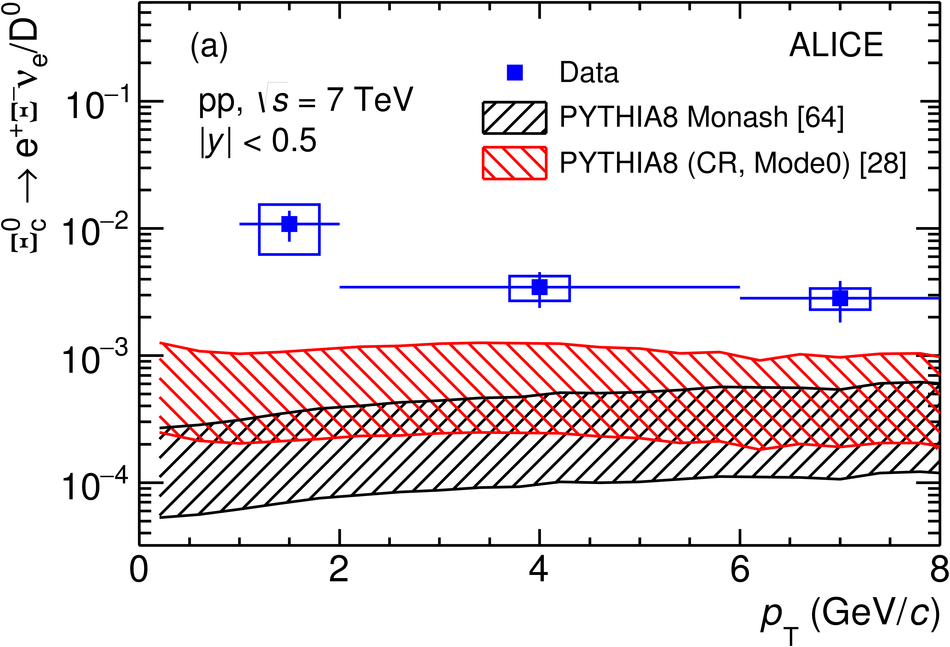# First measurement of $Ξ_{\rm c}^0$ production in pp collisions at $\mathbf{\sqrt{s}}$ = 7 TeV

The production of the charm-strange baryon $\Xi_{\rm c}^0$ is measured for the first time at the LHC via its semileptonic decay into e$^+\Xi^-\nu_{\rm e}$ in pp collisions at $\sqrt{s}=7$ TeV with the ALICE detector. The transverse momentum ($p_{\rm T}$) differential cross section multiplied by the branching ratio is presented in the interval 1 $<~$ $p_{\rm T}$ $<~$ 8 GeV/$c$ at mid-rapidity, $|y|$ $<~$ 0.5. The transverse momentum dependence of the $\Xi_{\rm c}^0$ baryon production relative to the D$^0$ meson production is compared to predictions of event generators with various tunes of the hadronisation mechanism, which are found to underestimate the measured cross-section ratio.

Figures

## Figure 1

 (a) Invariant-mass distribution of $\Xi^- \rightarrow \pi^-\Lambda$ (and charge conjugate) candidates integrated over \pt. The arrow indicates the world average $\Xi^-$ mass from Ref.~ and the dashed lines indicate the selected interval. (b) Invariant-mass distributions of right-sign and wrong-sign (and charge conjugate) pairs integrated over the whole \pt\ interval. The arrow indicates the \xicz\ mass~.## Figure 2

 Correlation between the generated \xicz-baryon \pt\ and the reconstructed \exipair\ pair \pt, obtained from the simulation based on {\sc pythia 6} described in the text.## Figure 3

 Product of acceptance and efficiency ($A\times\varepsilon$) of \xicz\ baryons generated in $|y|< 0.8$ decaying into ${\rm e}^+\Xi^-\nu_{\rm e}$ as a function of \pt, determined from simulations {\sc pythia} 6 (see text).## Figure 4

 Inclusive \xicz-baryon \pt-differential production cross section multiplied by the branching ratio into ${\rm e}^+\Xi^-\nu_{\rm e}$, as a function of \pt\ for $|y|< 0.5$, in \pp collisions at $\sqrt{s} =$ 7 TeV. The error bars and boxes represent the statistical and systematic uncertainties, respectively. The contribution from \xib\ decays is not subtracted.## Figure 5

 Ratio of the \pt-differential cross sections of \xicz\ baryons (multiplied by the branching ratio into ${\rm e}^+\Xi^-\nu_{\rm e}$) and \Dzero mesons~ as a function of \pt\ for $|y|< 0.5$, in \pp collisions at $\sqrt{s} =$ 7 TeV. The error bars and boxes represent the statistical and systematic uncertainties, respectively. Predictions from theoretical models, (a) {\sc pythia 8} with different tunes (b) {\sc dipsy}~ and {\sc herwig 7}~, are shown as shaded bands representing the range of the currently available theoretical predictions for the branching ratio of the considered \xicz\ decay mode.